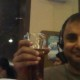# 50 functions to clear a basic interview for Business Analytics #rstats

Due respect to all cheat sheets and ref cards, but these are the functions that I use in a sequence to analyze a business data set.• Packages
1. install.packages(“Hmisc”)  installs package Hmisc
• Data Input
1. getwd() – Gets you the current working directory
2. setwd(“C:/Path”) -Sets the working directory to the new path , here C:/Path
3. dir() – Lists all the files present in the current working directory

here

a= read.csv (assigns the object name a to whatever comes to the right side)

You can also explicitly assign a character name to a data object using assign)

read.csv is a type of input command derived from read.table to read in a rectangular object (rows and columns)

header specifies whether the first line has variable names or not

sep denotes seperator (generally a comma for CSV files but can be space or tab for text files)

stringsAsFactors=T reads in strings as character values and not factor levels

• Object Inspection
1. str(a) Gives the structure of object named  including class, dimensions, type of variables , names of variables and a few values of variables as well. Only drawback is can throw up a lot of information for a big data set
2. names(a) Gives the names of variables of the object
3. class(a) Gives the class of a object like data.frame, list,matrix, vector etc
4. dim(a) Gives the dimension of object (rows column)
5. nrow(a) Gives the number of rows of object a- useful when used as an input for another function
6. ncol(a) Gives the number of columns of object a
7. length(a) Gives the length of object- useful for vectors, for a data frame it is the same as ncol
8. a[i,j] Gives the value in ith row and jth column
9. a\$var1 Gives the variable named var1 in object a . This can be treated as a seperate object on it’s own for inspection or analysis
• Data Inspection
1. head(a,10) gives the first ten rows of object a
2. tail(a,20) gives the last twenty rows of object a
3. b=ajay[sample(nrow(ajay),replace=F,size=0.05*nrow(ajay)),]

Lets get a 5 % random sample of the object ajay

[] uses the subset to give value in the specified row

Sample is the command for sample

So out nrow(ajay) or total number to be sampled of ,size= Size of sample it is taking 5% numbers, and these are the row numbers that are being returned. replace =F means each number is selected only once

• Math Functions

Basic-

1. sum  -sum
2. sqrt -square root
3. sd  -standard deviation
4. log –log
5. mean -mean
6. median– median

1. cumsum – Cumulative Sum for a column
2. diff –Differencing
3. lag – Lag
• Data Manipulation
1. paste(a\$Var) converts Var from Factor/Numeric variable to Charachter Variable
2. as.numeric(a\$Var2) Converts a character variable into a numeric variable
3. is.na(a) retruns TRUE wheneve it encounters a Missing Value within the object
4. na.omit(a) Deletes all missing values (denoted by NA within R)
5. na.rm=T (this option enables you to calculate values Ignoring Missing Values)
6. nchar(abc) gives the values of characters in a character value
7. substr(“ajay”,1,3) gives the sub string from starting place 1 to ending place 3. Note in R index starts from 1 for first object
• Date Manipulation

library(lubridate)
> a=”20Mar1987″
> dmy(a)
 “1987-03-20 UTC”
> b=”20/7/89″
> dmy(b)
 “1989-07-20 UTC”
> c=”October 12 93″
> mdy(c)
 “1993-10-12 UTC”

• Data Analysis
1. summary(a) Gives summary of object including min,max,median,mean, 1st quartile, 3rd Quartile) for numeric objects and frequency analysis of Factor variables
2. table(a) Gives Frequency Analysis of variable or obejct
3. table(a\$var1,a\$var2) Gives cross tabs of Var1 with respect to Var 2 of object a

library(Hmisc) loads HMisc which enables use to use describe and summarize function

1. describe(a\$var1) gives a much more elaborate and concise summary of the variable Var 1- it’s a better version of summary
2. summarize(a\$var1,a\$var2,FUN)  applies a function (like sum, median, summary or mean) on Var 1 , as GROUPED by Var2
3. cor(a) gives corelation between all numeric variables of a
• Data Visualization
1. plot(a\$var1,a\$var2) Plots Var 1 with  Var 2
2. boxplot(a) boxplot
3. hist(a\$Var1) Histogram
4. plot(density(a\$Var1) Density Plot
5. pie (pie chart)

Modeling

1. a=lm(y~x) creates model
2. vif(a) gives Variance Inflation  (library(car) may help)
3. outlierTest(a) gives Outliers

summary(a) gives model summary including parameter estimates

• Write Output
1. write.csv(a) Write output as a csv file
2. png(“graph.png”) Write plot as png file
3. q() –Quits R Session

——————————————————————————————————————————————————-

R is a huge language with 5000 packages and hundreds of thousands of functions.

But if you memorize these functions ~50, I assure you will make a much more positive impression in your business analytics interview !

Bonus-

```Sys.time() and Sys.Date() gives current   time and date (note the change in case)
while system.time(experession gives time taken to evaluate an expression)

Citation- http://www.ats.ucla.edu/stat/r/faq/timing_code.htm

Also

yo=function(a,b){ a*b*12} creates a custom function called yo which can be then invoked as yo(2,3)

Coming up-

the apply family, the Hadley collective  and GUIs  for the second round of interview ;)```## Author: Ajay Ohri

1.khushboo30jain says:
2.dataratta says: